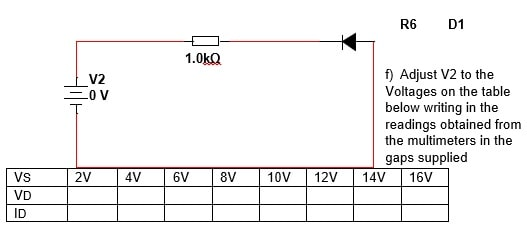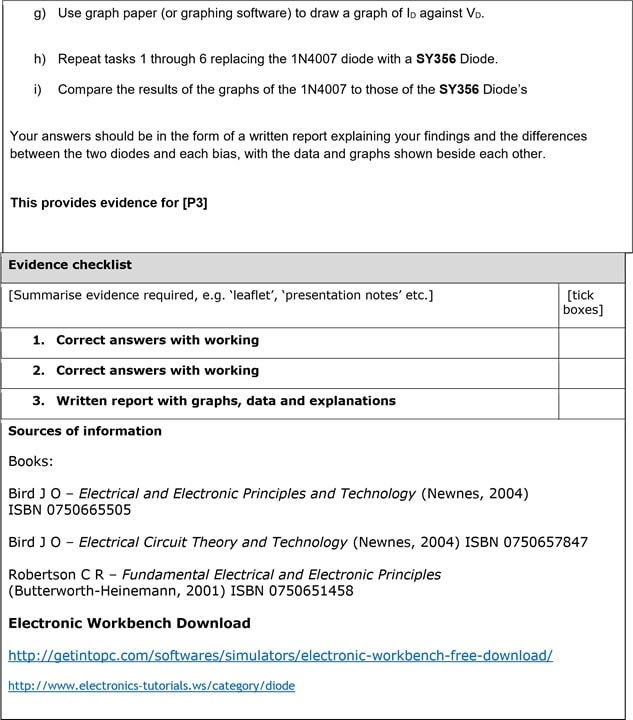# DC Networks Assignment 1

```{`Assignment brief – QCF BTEC
Qualification BTEC Level 3 Engineering
Unit number and title Unit 06 Electrical and Electronic Principles
Assignment title D.C. Networks – Assignment 1
`}```

In this assessment you will have opportunities to provide evidence against the following criteria. Indicate the page numbers where the evidence can be found.

 Criteria reference To achieve the criteria the evidence must show that the learner is able to: P1 Use DC circuit theory to calculate current, voltage and resistance in DC networks P3 compare the forward and reverse characteristics of two different types of semiconductor diode M1 use Kirchhoff’s laws to determine the current in various parts of a network having four nodes and the power dissipated in a load resistor containing two voltage sources

Purpose of this assignment

During this assignment you will need to produce evidence that is sufficient to meet the assessment criteria for P1, P3 and M1 for this unit.

Scenario

You have been asked by the electrical workshop technician to check and test, circuits used in the workshop before they are used. You have been asked to check basic circuits both by use of theory and practical.

All working out must be clearly shown; merely giving an answer will not meet the pass criteria. Both circuit 1a and circuit 1b need to be completed.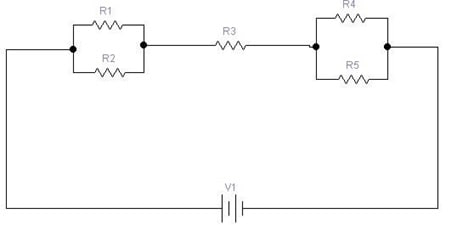Using circuit reduction techniques on the above circuit.

1. Reduce the circuit to the equivalent of three series resistors and redraw this new circuit.
2. Calculate the circuit’s total resistance and the current drawn from the supply.
3. Calculate the voltages across the three ‘series’ resistances.
4. Return to the original circuit and calculate the current flowing through the R1 resistor and the current flowing through the R2 resistor.
5. Calculate the current flowing through the R4 resistor and the current flowing through the R5 resistor.

Values for R1-R5 and V1 are different for each student and are listed at the end of this brief.

All working out must be clearly shown; merely giving an answer will not meet the pass criteria.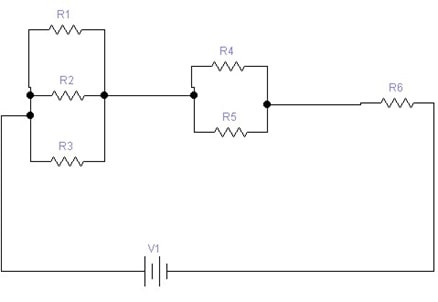Using circuit reduction techniques on the above circuit.

1. Reduce the circuit to the equivalent of three series resistors and redraw this new circuit.
2. Calculate the circuit’s total resistance and the current drawn from the supply.
3. Calculate the voltages across the three ‘series’ resistances of your redrawn circuit.
4. Return to the original circuit shown above and calculate the current flowing through the R1, the R2 and the R3 resistors.
5. Calculate the current flowing through the R4 resistor.
6. Calculate the current flowing through the R5 resistor.

Values for Voltage (V1) and Resistors (R1 through R5) can be found in the assessment plan. Make sure you use only the values next to your name.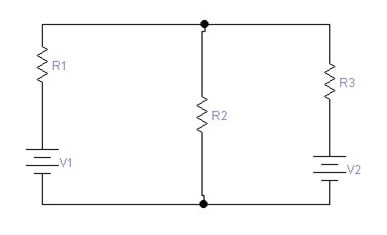Using Kirchhoff voltage and current laws and the values listed in the assessment plan, calculate the current flowing in each branch of the above diagram.

You must clearly show all working and each step taken in order to get the grade.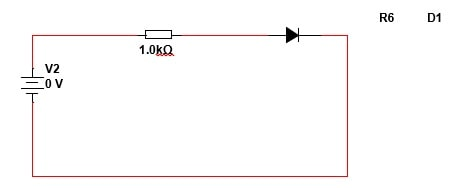```VS 	0.1V 	0.2V 	0.3V 	0.4V 	0.5V 	0.6V 	0.7V 	0.8V 	0.9V 	1.0V 	1.1V 	1.2V